Courses

# Micro- and Macro States - Statistical Ensembles, CSIR-NET Physical Sciences Physics Notes | EduRev

## Physics for IIT JAM, UGC - NET, CSIR NET

Created by: Akhilesh Thakur

## Physics : Micro- and Macro States - Statistical Ensembles, CSIR-NET Physical Sciences Physics Notes | EduRev

The document Micro- and Macro States - Statistical Ensembles, CSIR-NET Physical Sciences Physics Notes | EduRev is a part of the Physics Course Physics for IIT JAM, UGC - NET, CSIR NET.
All you need of Physics at this link: Physics

Microstates and Macrostates

Take-home message: The properties of a macrostate are averaged over many microstates.

Classical thermodynamics describes macroscopic systems in terms of a few variables (functions of state): temperature, pressure, volume... But such a system is really made of atoms, so a much richer description must be possible in principle: we could specify the quantum state of all the atoms--the microstate. Of course as the atoms interact this state changes very rapidly-perhaps 1035 times a second. But the observed macrostate doesn't change. Many different microstates all correspond to the same macrostate.

This suggests we can calculate the macroscopic behaviour of the system by averaging over the corresponding microstates. We can derive thermodynamics from the quantum behaviour of atoms and molecules.

Of course only a few systems are really fully calculable: those in which the interactions between atoms are particularly simple--or absent. We will be able to tackle the ideal paramagnet and the ideal gas, including the latter at densities so high that the quantum nature of the system becomes important. The van der Waals gas and a paramagnet with nearest neighbour interactions can be worked out, as can crystalline solids with simple defects. Going beyond that is tough. But the proof-of-principle that these give is compelling.
The crucial link from microscopic to macroscopic properties is as follows. If the value of some quantity X in the i th microstate is Xi, and the probability that the system is in that microstate is pi, then the value of X in the macrostate is the ensemble average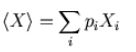We start by considering an isolated system (constant energy, volume and particle number). The fundamental principle that allows the averaging over microstate to be done is the postulate of equal a prioriprobabilities or, in plain English, the assumption that all allowed microstates are equally likely. (Allowed or accessible means having the same volume, particle number and and total energy as the macrostate.) We use Ω for the number of such microstates, so the probability of the system being in any one microstate is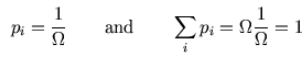Simple example

Microstates

Imagine we have counters, blue on one side and green on the other, and we toss them and place them on a 6x6 checkerboard. Full information involves listing the colour at each site: this is the equivalent of a microstate.

Many different patterns are possible, such as the following. Every configuration is equally likely--or unlikely--to occur: There are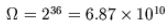patterns and the the probability of each is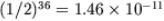. (This satisfies the ``postulate of equal a priori probabilities''.)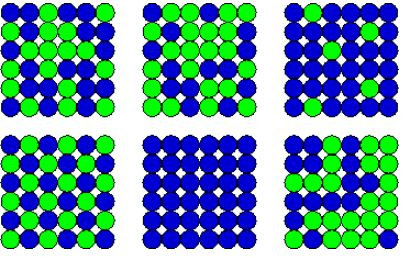Suppose from a distance we only knew how many counters were green and how many blue, without being able to distinguish different arrangements of the same numbers of counters. Then a ``macrostate'' would be characterised simply by the total number of green counters (the rest being blue).

Clearly, most macrostates correspond to many microstates. If the macroscopic description is ``15 green'', the following are a few of the allowed microstates: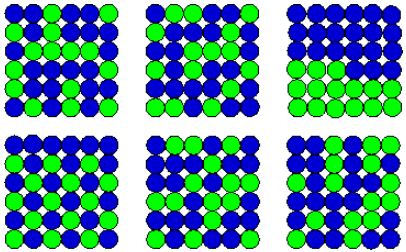How many are there in total? This is the common problem of splitting a group of N into two smaller groups, of n and N - n, without caring about the ordering in each group, and the number of ways of doing it is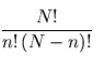Think of counters on squares: there are N! ways of putting N distinguishable counters on N squares. However if n of the counters are green, there are n! ways of arranging the green counters among themselves without changing the pattern, and (N - n)! ways of arranging the blues.
Here, N = 36 and n = 15, so the total is 5.59x109. For n = 10 there are only 2.54x108, whereas for n = 18, there are 9.08x109. This is the maximum.

The numbers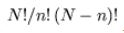are called the binomial coefficients (since they enter the binomial expansion) and they are written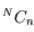or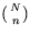.

159 docs

,

,

,

,

,

,

,

,

,

,

,

,

,

,

,

,

,

,

,

,

,

,

,

,

;